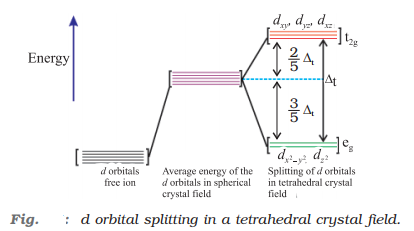According to Werner’s theory of coordination compounds, there are two types of valencies in coordination compounds:

• Primary valencies: These are ionizable valencies, satisfied by anions and determines the charge on the complex ions.
• Secondary valencies: These are non-ionisable valencies, satisfied by ligands and determines the coordination number of the metal atom.

# Valence Bond Theory

Valence bond theory states that the metal atom or ion under the influence of ligands can use its (n-1)d, ns, np or ns, np, nd orbitals for hybridisation to yield a set of equivalent orbitals of definite geometry such as octahedral, tetrahedral, square planar and so on. These hybridised orbitals are allowed to overlap with ligand orbitals that can donate electron pairs for bonding.

## Thus the basic principles involved in the valence bond theory are:

• Hybridisation of orbitals
• Bonding between the ligands and the metal atoms/ion.
• Relation between the observed magnetic moment and the bond type.

## Limitation of the Valence Bond theory

• It does not tell anything about the spectral properties of the complexes.
• It does not give quantitative interpretation of magnetic data.
• It does not distinguish between strong and weak ligands.
• It does not explain the colour exhibited by coordination compounds.
• It does not give a quantitative interpretation of the thermodynamic or kinetic stabilities of coordination compounds.

# Crystal Field Theory

In crystal field theory (CFT), ligands are considered as point charges and the interaction between the ligands and the metal ion is purely electrostatic in nature. The five d-orbitals in an isolated gaseous metal atom/ion have same energy, i.e., they are degenerate. The degeneracy is lost in the presence of the ligand field.

The five d-orbitals are classified as:

• Three d-orbitals, dxy, dyz and dzx that are oriented in between the coordinate axes and are called t2g orbitals.
• Two d-orbitals, dx2 ‒ y2 and dz2 that are oriented along the x – y axes and are called eg – orbitals.

## Factors affecting the splitting of d-orbitals:

• Nature of the ligand
• Nature of the metal ions
• Geometry of complex whether it is octahedral or tetrahedral
• Oxidation state of the metal ion

## Crystal field splitting in octahedral complexes

• Here energy of eg set of orbitals > energy of t2g set of orbitals.
• Ligands for which energy separation, Δo < P (the pairing energy, i.e., energy required for electron pairing in a single orbital) form a high spin complex.
• Ligands for which energy separation, Δo > P, form low spin complex.## Crystal field splitting in tetrahedral complexes

• Here energy of t2g set of orbitals > Energy of eg set of orbitals.
• In such complexes d-orbital splitting is inverted and is smaller as compared to the octahedral field splitting.
• No pairing of electrons is possible due to the lowest splitting energies which leads to high spin complexes.